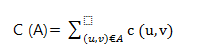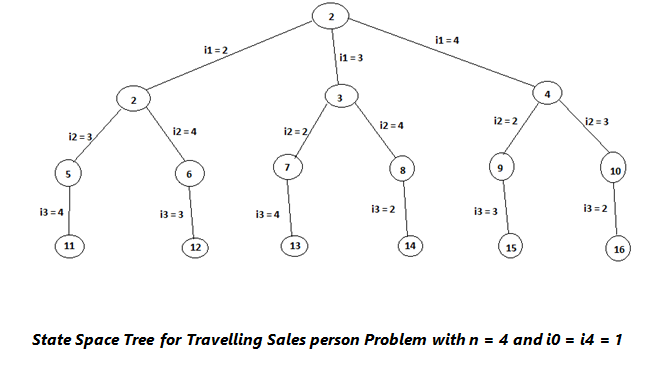# Travelling Salesman Problem

In this article, we will learn about the travelling salesman problem and prove that travelling salesman problem is the NP complete problem.
Submitted by Shivangi Jain, on July 29, 2018

## Travelling Salesman problem

This problem can be stated as- "Given n number of cities and a travelling salesman has to visit each city. Then we have to find the shortest tour so that the travelling salesman can visit each and every city only once."

This travelling salesman problem is one of the examples of NP-Complete problems.

In the travelling salesman problem, we are given a complete undirected graph G = (V, E) that has a non-negative integer cost c (u, v) associated with each edge (u, v) belongs to E and we must find a tour of G with minimum cost.

Let C (A) denotes the total cost of the edges in the subset A is the subset E.Practically, it is always cheapest to go directly from a place w, going by way of any intermediate stop V can’t be expensive. Or say, cutting out an intermediate stop never increase the cost. This can be formalized that the cost function c satisfies the triangle inequality, if for all vertices u, v, w £ V .

C (u, w) <= c (u, v) + c (v, w)

This triangle inequality is natural one, and is many application it is automatically satisfied. In this problem, our tour starts from an initial state and completes after returning to original state passing through all intermediate states.

If the graph has n vertices, i.e., |V| = n, then the solution space S is given by S = { 1, π, 1, π: is a permutation of (2, 3, ..., n)}.

Then |S| = (n-1)!

The size of S can be reduced by restricting S so that (1, i1,...i2,i(n-1), 1) belongs to S if and only if (ij, ij + 1) £ E, 0 <= j <= n-1, and i0 = in = 1.

State space tree for this problem, for n = 4 and initial and final states 1.